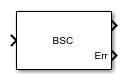# Binary Symmetric Channel

Introduce binary errors

• Library:
• Communications Toolbox / Channels

•## Description

The Binary Symmetric Channel block introduces errors to the input signal transmitted through a binary symmetric channel. The errors are introduced based on the specified Error probability. For more information, see Tips.

## Ports

### Input

expand all

Input signal, specified as a column vector or an NS-by-NC matrix of `Boolean` values. NS is the number of samples per channel. NC is the number of independent data channels. For more information, see Tips.

### Output

expand all

Binary output signal, returned as a column vector or matrix with the same dimensions as `Input`. The output signal is a version of the input signal that has been modified by introducing random errors based on the specified Error probability. To set the output data type, use Output data type.

Error locations, returned as a column vector or matrix with the same dimensions as `Input`. Element values in `Err` are `1` or `0`, where:

• `1` indicates that the corresponding element in `Output` has an error.

• `0` indicates that the corresponding element in `Output` does not have an error.

The data type of `Err` is the same as Output, as set by Output data type.

#### Dependencies

To enable this port, select Output error vector.

## Parameters

expand all

Probability of error occurrence for the input signal elements, specified as a scalar in the range [0,1]. The probability of error applies independently for each element.

To enable the `Err` output port to the block, select this parameter.

Select the output data type as `double`, `single`, or `boolean`. This parameter sets the output data type for both the Output and Err ports.

Initial seed value for the random number generator used by the block, specified as an integer. The block uses the `mt19937ar` algorithm to generate uniformly distributed random numbers. For details about the `mt19937ar` algorithm, see Creating and Controlling a Random Number Stream.

Type of simulation to run, specified as:

• `Code generation` –– Simulate the model using generated C code. The first time you run a simulation, Simulink® generates C code for the block. The C code is reused for subsequent simulations as long as the model does not change. This option requires additional startup time.

• `Interpreted execution` –– Simulate the model using the MATLAB® interpreter. This option shortens startup time. In `Interpreted execution` mode, you can debug the source code of the block.

## Block Characteristics

 Data Types `Boolean` | `double` | `fixed point` | `integer` | `single` Multidimensional Signals `no` Variable-Size Signals `no`

## Tips

• When the input consists of not `Boolean` values, Binary Symmetric Channel converts zero-valued elements to 0 and converts nonzero-valued elements to 1.

• The Binary Symmetric Channel block creates and uses an independent `RandStream` to provide a random number stream for probability determination.

• To generate repeatable results, use the same Initial seed value.

• To generate independent probability statistics, set different Initial seed values for multichannel signals, multiple processing chains, or simulation runs.

## Version History

Introduced before R2006a

expand all

Behavior changed in R2018b